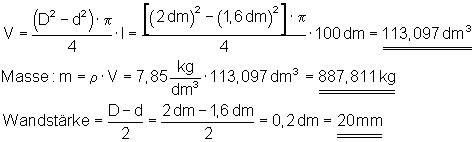# What is the formula for determining the mass?

## Exercises Volume and Mass I

First, I will again introduce the formula for calculating the mass of a body:

To calculate the mass of a body we need the density and the volume:

Mass = volume times density.### 1. Calculate the volume and mass of the complex casting!

Density:### 2. Calculate the volume and mass of the aluminum part!

Density:### 3. Calculate the volume and mass of the steel part.

Density:### 4. Calculate the volume and mass of the copper part!

The material is 12 mm thick.

Density: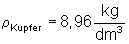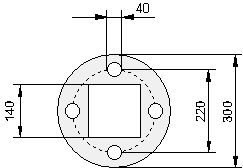### 5. A steel pipe is 10 m long (L = 10 m), has an outside diameter of D = 20 cm and an inside diameter of d = 160 mm.

Calculate that volume, the Dimensions and the Wall thickness of the pipe!### 1. Detailed solution:

Calculate the volume and mass of the casting.

Density: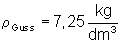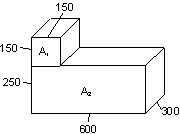All dimensions in the drawing are converted to decimeters (dm).
The following applies for the conversion: 100 mm = 1 dm, 10,000 mm2 = 1 dm2 and 1,000,000 mm3 = 1 dm3
To determine the volume, the area of ​​the workpiece is multiplied by its thickness. The results are to be rounded to three places behind the decimal point.### 2. Detailed solution:

Calculate the volume and mass of the aluminum part.

Density:All dimensions in the drawing are converted to decimeters (dm).
The following applies for the conversion: 100 mm = 1 dm, 10,000 mm2 = 1 dm2 and 1,000,000 mm3 = 1 dm3
To determine the volume, the area of ​​the workpiece is multiplied by its thickness. The results are to be rounded to three places behind the decimal point.#### 3. Detailed solution:

Calculate the volume and mass of the steel part.

Density: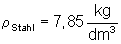All dimensions in the drawing are converted to decimeters (dm).
The following applies for the conversion: 100 mm = 1 dm, 10,000 mm2 = 1 dm2 and 1,000,000 mm3 = 1 dm3
The results are to be rounded to three places behind the decimal point.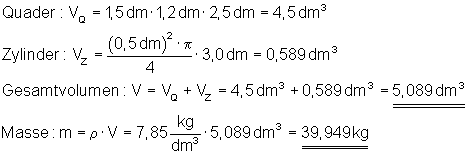### 4. Detailed solution:

Calculate the volume and mass of the copper part. The material is 12 mm thick.

Density:All dimensions in the drawing are converted to decimeters (dm).
The following applies for the conversion: 100 mm = 1 dm, 10,000 mm2 = 1 dm2 and 1,000,000 mm3 = 1 dm3
To determine the volume, the area of ​​the workpiece is multiplied by its thickness. The results are to be rounded to three places behind the decimal point.### 5. Detailed solution:

A steel pipe is 10 m long (L = 10 m), has an outside diameter of D = 20 cm and an inside diameter of d = 160 mm.
Calculate the volume, mass and wall thickness of the pipe.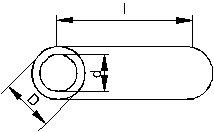All dimensions in the drawing are converted to decimeters (dm).
The following applies for the conversion: 100 mm = 1 dm, 10,000 mm2 = 1 dm2 and 1,000,000 mm3 = 1 dm3
The results are to be rounded to three places behind the decimal point.